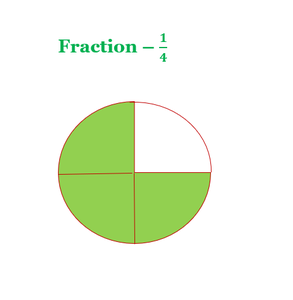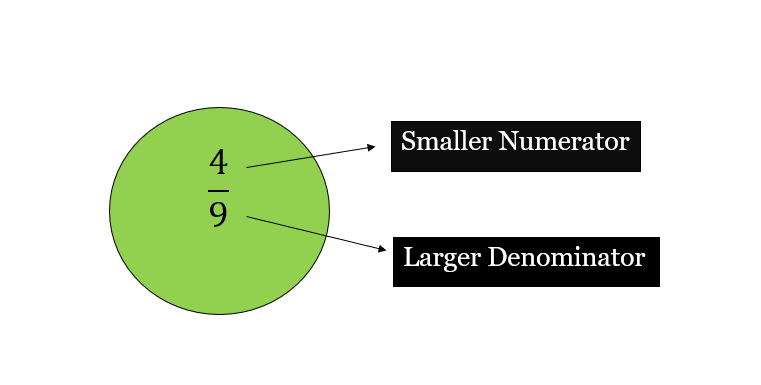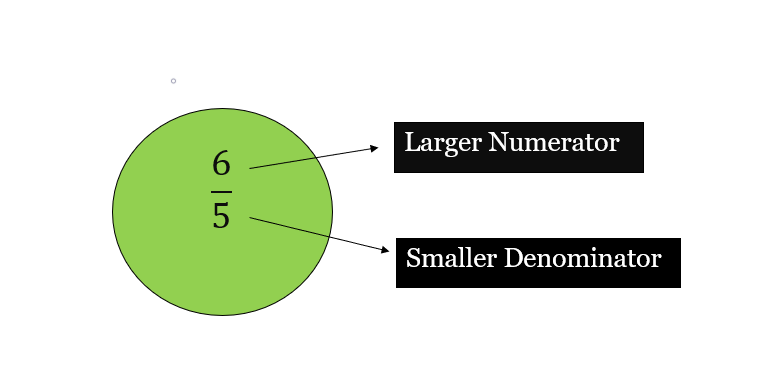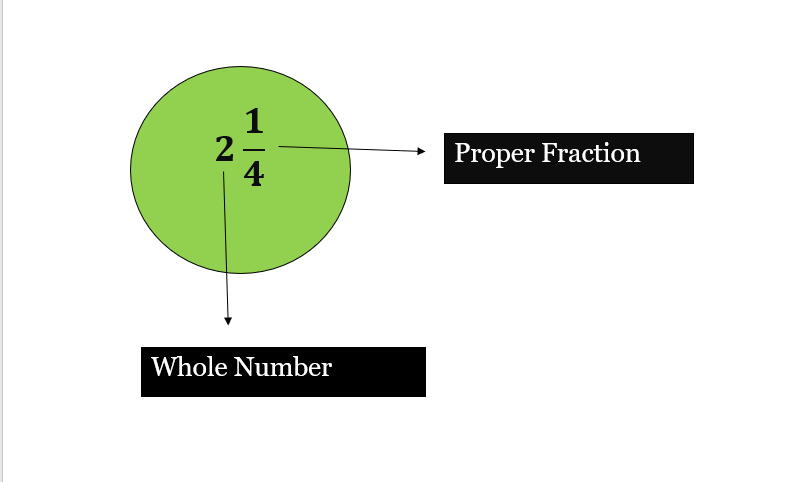# What is a fraction?

• Last Updated : 22 Sep, 2021

The method to represent and work with numbers is known as the number system. A number system is a system of writing to represent numbers. It is the mathematical notation used to represent numbers of a given set by using digits or other symbols. It has arithmetic operations to perform division, multiplication, addition, and subtraction between numbers. Some important number systems are the decimal number system, binary number system, octal number system, and hexadecimal number system.

### Numbers and Digits

Numbers are the counts or measurements used in mathematics, numerals are used to define numbers. A numeral can be defined as a symbol used for counting, for instance, there are 55 books in the library, where 56 is the numeral which is a combination of digits 5 and 6. A digit is a single numeral, the combination of digits form numerals. In the decimal number system, there are 10 digits, they are 0, 1, 2, 3, 4, 5, 6, 7, 8, 9.

Attention reader! All those who say programming isn't for kids, just haven't met the right mentors yet. Join the  Demo Class for First Step to Coding Coursespecifically designed for students of class 8 to 12.

The students will get to learn more about the world of programming in these free classes which will definitely help them in making a wise career choice in the future.

### Naturals Numbers

In the Number system, Natural numbers are the numbers that are starting from 1, and counts up to Infinity are called Natural Numbers. For example – (1, 2, 3, 4, 5, 6, 7, 8, 9, 10, 11,12, 13, 14, 15, 16, 17, 18, 19, 20, 21… ∞) are Natural Numbers.

### What is a fraction?

A fraction is a number that is used to represent a whole number that has been divided into equal parts. A fraction contains two parts i.e. numerator and denominator. The number present on the top is known as the numerator and the number present on the bottom is known as the denominator. The numerator part defines how many parts of the whole are there, whereas the denominator defines the total number of equal parts in a whole.For Example – 1/10 is a fraction in which 1 is a numerator and 10 is a denominator, another example can be 15/23 where 15 is the numerator and 23 is the denominator.

Different types of fractions

There are majorly four types of fractions. They are unit fractions, proper fractions, improper fractions, and mixed fractions. Let’s learn about all the four types in detail:

Unit Fraction

A unit fraction is defined as a fraction with 1 as a numerator is known as a Unit Fraction. For Example – 1/8, 1/10, 1/4, 1/6, 1/11, etc. It can be said that all unit fractions are proper fractions since all unit fractions have 1 in the numerator, which is lesser than the denominator.

Proper Fraction

A Proper fraction is defined as a fraction in which the numerator value is less than the denominator value then it is known as a proper fraction. For Example 4/9, 1/10, 2/5, 3/7, 5/9, etc.Improper Fraction

An Improper fraction is defined as a fraction in which the numerator value is greater than the denominator value, then it is known as an improper fraction. For Example 6/5, 11/10, 11/5, 5/3, 2/1, etc.Mixed Fraction

A Mixed fraction consists of a whole number with a proper fraction then it is known as a mixed fraction. For Example, if 2 is a whole number and 1/4 is a fraction then 2¼ is a mixed fraction.Other Types Of Fractions

• Like Fractions

Two or more than two fractions whose denominators are the same are known as like fractions. Examples of like fractions are,

1. 2/9, 3/9, 5/9, 9/9, 4/9 (Here, the denominators of all the fractions are the same that is 9)
2. 3/10, 7/10, 1/10, 9/10, 6/10 (Here, the denominators of all the fractions are the same that is 10)
3. 1/7, 2/7, 4/7, 5/7, 7/7, 8/7
4. 1/2, 7/2, 6/2, 5/2, 9/2
5. 7/5, 1/5, 4/5, 3/5
• Unlike Fractions

The fractions with different denominators are known as, unlike fractions. Example of unlike fraction,

1. 9/2, 1/6, 5/4, 1/2, 7/3 (Here, the denominator of all the fractions are different)
2. 1/2, 1/4, 2/3, 5/6, 8/9 (Here, the denominator of all the fractions are different)
3. 3/8, 2/3, 3/5, 2/7, 1/3
4. 1/9, 2/7, 3/4, 2/5, 7/4, 3/2
5. 4/2, 1/6, 2/3, 7/5
• Equivalent Fractions

Equivalent fractions are defined as those fractions which result in the same value after simplification then they are equivalent to each other.

### Sample Problems

Question 1: How to determine whether fractions are equivalent?

First, it is needed to simplify the given fractions to find whether they are equivalent fractions or not. Suppose we have two fractions 3/9 and 2/3. 3/9 can be further simplified to 1/3, but 1/3 is not equal to 2/3. Therefore, they are not equivalent fractions. But, 5/6 and 10/12 are equivalent fractions because after simplification of 10/12 we get 5/6 which is equal to 5/6. For example of equivalent fraction, 2/3 and 4/6 are equivalent fractions because 4/6 = (2 × 2)/(2 × 3) = 2/3 (they are equivalent to each other)

Question 2: Why fractions are used?

Fractions tell us the portion of a whole things. For example, if we divide a pizza into 8 equal parts and we take one piece, this mean that 1/8 of the cake is eaten and 7/8 is left.

Question 3: What type of fraction is this – 1/2, 1/5, 1/7, 1/10, 1/3?

This is a Unit fraction because all the fractions have 1 as a numerator.

Question 4: Determine the type of the fraction 7¼?

Mixed fraction because it is a combination of both whole number and a proper fraction in which 7 is the whole number and 1/4 is the proper fraction.

Question 5: What is the equivalent fraction of 3/5?

To find the equivalent fraction of 3/5, we need to multiply both numerator and denominator by the same number. Hence, (3/5) × (2/2) = 6/10. Therefore equivalent fraction of 3/5 is 6/10.

Question 6: What is the equivalent fraction of 7/5?

To find the equivalent fraction of 7/5, we need to multiply both numerator and denominator by the same number.

Hence, ( 7/5 ) × ( 2/2 ) = 14/10

Therefore equivalent fraction of 7/5 is 14/10.

Question 7: What are the equivalent fractions of 5/10?